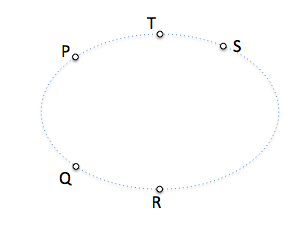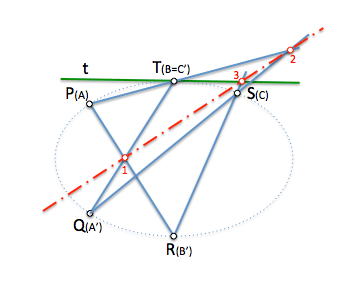# Geometría proyectiva: Application of overlapping series of second orderProjective concepts that we have developed in studying the overlapping series of second order, whose base is a conical, They allow to solve problems of determination of tangent points of a Conic defined by five points or five restrictions through the combination of points and tangents with their respective points of tangency.

To solve this type of problems we will remember that given two sets of second order, by projecting them from two homologous elements are obtained do perspective that are cut in the projective axis series (Straight from Pascal). In the following figure, rays counterparts a-a.’ They determine the double beam of beams perspective, While the b-b’ and c-c’ cut them into paragraphs 1 and 2 perspective axis respectively (“and” It is cited projective series shaft)#### General model for the Pascal line

The homologous points that serve as vertices for these beams perspective can be any of the three pairs that define the is among the series of second order. We can see that if we project from all of them we get three points (1,2 and 3) step of the Pascal line, It will cut to the conical double points series (It will be imaginary if this straight line is exterior to the conical).

#### Pascal-straight with a tangent

The exposed projective model allows to relate the conical with its tangent points, thinking that a tangent is a rope of the Conic whose ends match. For example, If we move the point “C’” the previous figure to match point “B” keeping the geometric constraints of this figure, We will have to b-c’ It has become a tangent that will follow containing the point “3” projective shaft.

#### Pascal straight with two tangents

Matching a second pair of points as the a-b’ We will obtain a variant of the previous model, but in this case with two tangents.

#### Pascal straight with three tangent

If we do agree the two points that are free, C-A ’, We will have the third tangent.

### Statement of issues

These figures allow us to pose problems of determination of tangents at the points of the Conic as we will see in a couple of examples, the reader leaving the resolution of the remaining.

The problems that can arise, understanding the Conic as a set of points, its:

1. Given five points of a conical, determine the tangent one.
2. Given a tangent with your point of contact and three additional points of a conical, determine the tangent at another point.
3. Given two tangent with their respective contact points and an additional point, determine the tangent at that point.

### Application to problem solving

We will resolve the first of the problems raised as an example:

Dice points P, Q, R, S and T belonging to a conical, determine the tangent at the point “T“.#### 1.-Determination of the figure of analysis of application

We will use as a figure of analysis to solve the problem that we have tagged as “Pascal-straight with a tangent”, as in this variant of the “General model” We have a tangent.#### 2.- Allocation of the corresponding labels

We shall first proceed to identify the points of the wording of the problem with the figure of analysis, taking into account that, in this case, We will have to assign a point from each series of second order point “T” in which we want to find the tangent.#### 3.- Determination of the is

Once determined the elements of the series, We obtain the projective axis of the same (Straight from Pascal).#### 4.- Resolving the problem

Will finally determine the tangent knowing that this, Ray b-c ’, cut in the projective shaft with his counterpart Ray c’Similarly, we solve the two remaining cases.

Can you solve them?